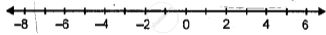# Fill in the Blanks, Using the Following Number Line: – 4 is _______ than -8 and 4 is _______ than 8. - Mathematics

Fill in the Blanks

Fill in the blanks, using the following number line:– 4 is _______ than -8 and 4 is _______ than 8.

#### Solution

– 4 is greater than -8 and 4 is less than 8.

Is there an error in this question or solution?

#### APPEARS IN

Selina Class 6 Mathematics
Chapter 7 Number Line
Exercise 7 (A) | Q 1.5